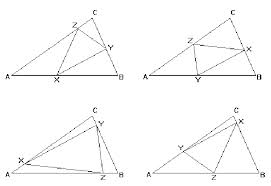# Triangle in Triangle

Geometry Level 5Find The maximum possible perimeter of an triangle (XYZ) which is inscribed in an another acute angled Triangle(ABC). And It is given that circum-radius of outer Triangle is R

DETAILS AND ASSUMPTIONS

$\bullet$ Inner Triangle XYZ (as shown above) must touches the all 3 sides Of Outer Triangle ABC

$\bullet$ Use R=1

×